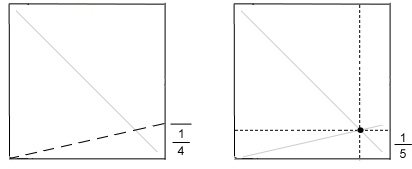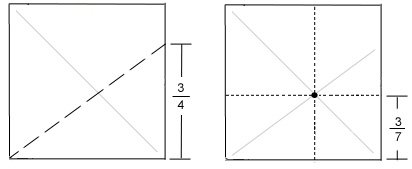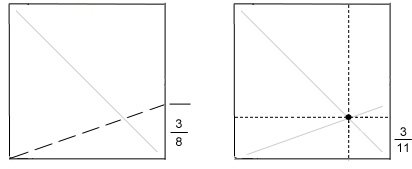# Divide Paper into nths Divisions

For the most part, knowing how to divide a sheet of paper into equal thirds, fourths, or fifths is enough. On a rare occasion you may need to divide a sheet into equal sevenths, or ninths. Divisions smaller than that are quite specialized and few “everyday” paper folders will need that type of skill.

However, if you are one of those people who enjoy origami and math, or if you design your own origami, you may wish to learn how to divide a square sheet of paper into any number of divisions. It is surprisingly easy – you only need to know 3 things:
• the General Idea,
• the Powers of Two, and
• the Methodology

#### Divide Paper into nths Divisions: The General Idea

The general idea is this: you will make two diagonal folds and the intersection of the two folds is your target division.

The first diagonal fold is easy: fold in half corner to corner and then unfold. The second fold requires a bit of calculation and an understanding of the “power of two”.The Powers of Two

The “power of two” simply means:
two to the power of one equals two,
two to the power of two equals four,
two to the power of three equals eight,…This can be summarize in a table ————>
 21 = 2 22 = 4 23 = 8 24 = 16 25 = 32

The Methodology

To determine the location of the second diagonal fold you need to find the greatest “power of two” that is less than the number of divisions you want (n).

So for example, if you want to divide a square sheet of paper into 5 equal segments then n = 5.The greatest “power of two” that is less than 5 is 4, as shown on the table.The difference between 5 and 4 is 1.

The distance from the bottom edge of the paper should be 1/4.

 21 =  2 22 =  4 23 =  8 24 = 16 25 = 32

Fold your second diagonal from the bottom-left corner to the 1/4 mark; the intersection (dot) is the location of the 1/5 division.The sequence of folds to achieve 1/5 division is shown here. Notice that finding the 1/4 mark along the right edge of the paper is easy (fold in half, then fold in half again). Indeed, all the “powers of two” are easy to obtain because folding a sheet of paper in half repeatedly will give you 2, 4, 8, 16, 32,… divisions.

This method of finding intersecting diagonals can be done with any number of divisions.

Let’s try another example. You wish to divide a square sheet of paper into 7 segments.

The greatest “power of two” less than 7 is, again, 4.7 subtract 4 is 3.

So the vertical-distance from the bottom-edge of the paper should be 3/4.

The second diagonal crease should extend from the bottom-left corner to the 3/4 mark on the right edge of the paper; the intersection (dot) of the two diagonal creases marks the 3/7 division.

 21 =  2 22 =  4 23 =  8 24 = 16 25 = 32You can then divide the 4/7 section into 1/7ths (fold section in half and then half again). Use the 1/7th to further divide the 3/7 section into sevenths. This method can also be seen here.

Here’s one more example: divide a square sheet of paper into 11 equal segments.

The greatest “power of two” less than 11 is 8.

11 subtract 8 is 3.

So the vertical-distance from the bottom-edge of the paper should be 3/8.

Fold the second diagonal so the crease extends from the bottom-left corner to the 3/8 mark on the right edge of the paper; the intersection (dot) of the two diagonal creases marks the 3/11 division.

 21 =  2 22 =  4 23 =  8 24 = 16 25 = 32This video by Tadashi Mori gives a great explanation as to why this works.